# Common Size Statement

Common Size Statement is a statement in which each item is presented in a percentage form. It could be a percentage of the total amount/ aggregate or revenue from operations. Because of this a common size statement  is also called a component percentage statement.

It can be defined as a money related statement which contemplates any key changes and variation patterns in the monetary position of the company. If one has to assess the changes in percentage over a period of time, a common size statement can be prepared for successive years. This will make the comparison of year to year easier and any changes will be visible in the form of percentage increase or decline.

A common site statement could be of a balance sheet, profit or loss or any other financial report.

For example, a common size balance sheet would show the percentage of each asset item to the total/ aggregate assets, and similarly all liabilities would be shown as percentage of total liabilities. In the case of a common size statement of profit and loss, the items of expenditure will be presented as a percentage of the revenue from operations.

### Use of Common Size Statement :

Common size statements come really handy if one wishes to compare enterprises which substantially differ in their size. Analysing financial figures in case of these enterprises would be a cumbersome task.

Also, common size statements also help in making inter-company comparisons or industry related comparisons.

### Format of Common Size Statement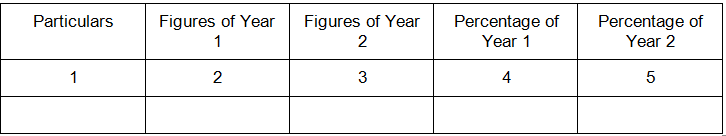### Examples-

Example 1-

Following is the information provided by C LTD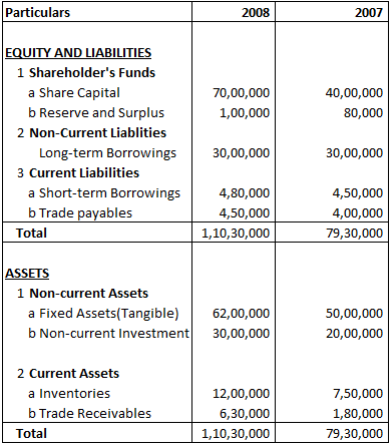Absolute Change and Percentage Change in share capital is?

Explanation: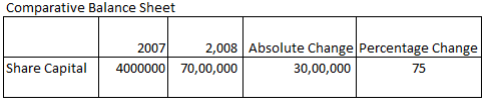Absolute Change = Current year’s gure – Previous year’s figure

= 7000000 – 4000000

= 3000000

Percentage Change = (Absolute Change/Amount of Previous Year) x 100

= (3000000/4000000) x 100

= 75 %

Example 2-

Following is the Balance sheet of CC Ltd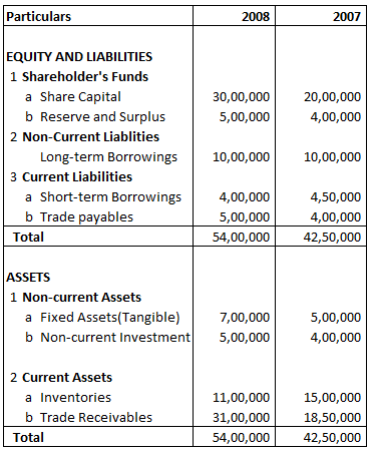Absolute Change and Percentage Change in Reserve and Surplus is?

Explanation: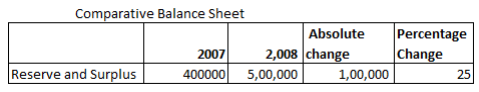Absolute change = Current year’s gure – Previous year’s figure

= 500000 – 400000

= 100000

Percentage Change = (Absolute Change/Amount of Previous Year) x 100

= (100000/400000) x 100

= 25

Example 3-

Following is the Balance sheet of AB Ltd.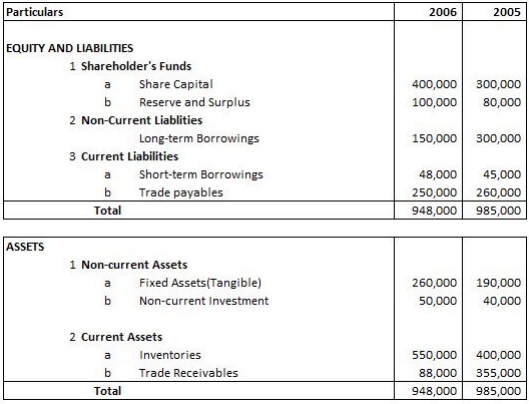Absolute Change and Percentage Change in Inventories is?

Explanation: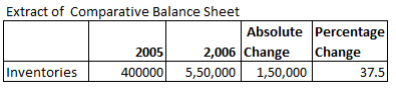Absolute Change = Current year’s gure – Previous year’s figure

= 550000 – 400000

= 150000

Percentage Change = (Absolute Change/Amount of Previous Year) x 100

= (150000/400000) x 100

= 37.5